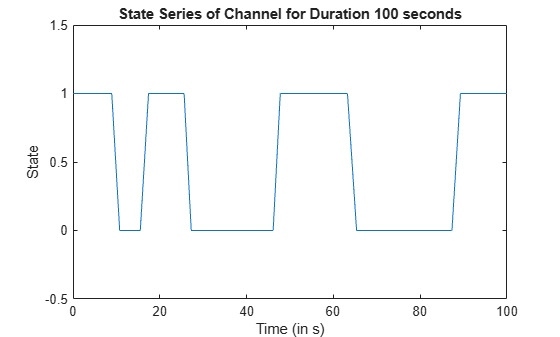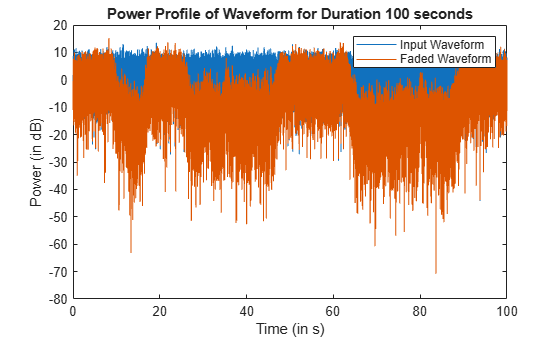# Simulate and Visualize a Land Mobile-Satellite Channel

This example shows how to model a two-state land mobile-satellite (LMS) channel model by generating a state series, its respective space series, and the channel coefficients. In a scenario involving a satellite terminal and a mobile terminal, a signal being transmitted through the channel does not always have an ideal line-of-sight path. In some cases, the signal experiences phenomena such as doppler shift, shadowing, and multipath fading. Appropriately modeling the effects of such phenomena is essential to properly design end-to-end communication links that are able to handle and compensate the effects of the channel. The example assumes an urban scenario and uses a carrier frequency of 3.8 GHz. This model is applicable for frequencies in the range 3 to 5 GHz.

### Introduction

An LMS channel model aims at simulating the channel envelope that is observed in a satellite-to-ground channel. Given the moving nature of the terminals, the channel envelope experiences variations due to movement of the transmitting and receiving terminals, blockage due to buildings and foliage, shadowing, and multipath.

This example models such a channel by using a two-state semi-Markov chain, where the channel alternates between a good and bad state. A good state is characterized by either line-of-sight conditions or partial shadowing conditions, whereas a bad state is characterized by either severe shadowing conditions or complete blockage.

The following block diagram shows the step-by-step procedure to model the channel:In addition to the environment and carrier frequency defined for this example, the modeling of the channel is done by setting up the scenario. This requires defining the following parameters:

• Elevation angle

• Velocity of the ground terminal

• Sampling time of the channel

• Azimuth orientation of the ground terminal

• Initial state of the channel

• Total simulation duration

### Environment Setup and Initial State Assignment

Set up the environment between the satellite terminal and the mobile terminal on the ground. Display the properties of the environment.

```% Carrier frequency in Hertz cfg.CarrierFrequency = 3.8e9; % Elevation angle with respect to ground plane in degrees cfg.ElevationAngle =45; % Speed of movement of ground terminal in meters per second cfg.Velocity =2; % Sampling interval in seconds cfg.SampleTime =0.0025; % Direction of movement of ground terminal in degrees cfg.AzimuthOrientation =0;```

Assign a suitable initial state for the model.

```cfg.InitialState ="Good"; % Total duration of channel modeling in seconds cfg.TotalSimulationTime =100; disp(cfg)```
``` CarrierFrequency: 3.8000e+09 ElevationAngle: 45 Velocity: 2 SampleTime: 0.0025 AzimuthOrientation: 0 InitialState: "Good" TotalSimulationTime: 100 ```

Obtain the respective LMS parameters using the configuration defined in ITU-R P.681-11 recommendation Section 3.1 Annexure 2 . The function `HelperGetLMSInputParams` carries out the necessary operation.

`[paramsGoodState,paramsBadState] = HelperGetLMSInputParams(cfg);`

Initialize random number generator with seed. Vary the seed to obtain different channel realizations. The default value 73 is an arbitrary value.

```seed = 73; rng(seed);```

### Channel Model

Model the LMS channel using the setup defined in the structure `cfg`.

If you have LMS data, you can update the fields of the `paramsGoodState` and `paramsBadState` structures, and then pass the structures to the `HelperModelLMSChannel` helper function.

`[stateSeries,channelCoefficients] = HelperModelLMSChannel(cfg,paramsGoodState,paramsBadState);`

### Channel Visualization

Plot the state series and its respective space series using the channel coefficients generated as a result of modeling.

```timeVector = 0:cfg.SampleTime:cfg.TotalSimulationTime; plot(timeVector,stateSeries) title(['State Series of Channel for Duration ' num2str(cfg.TotalSimulationTime) ' seconds']) axis([0 timeVector(end) -0.5 1.5]) xlabel('Time (in s)') ylabel('State')```Plot the space series to show how the instantaneous power of the channel envelope varies with time.

```figure(2) plot(timeVector,20*log10(abs(channelCoefficients))) title(['Space Series of Channel for Duration ' num2str(cfg.TotalSimulationTime) ' seconds']) xlabel('Time (in s)') ylabel('Path Gain (in dB)')```### Further Exploration

This example uses three structures: `cfg`, `paramsGoodState`, and `paramsBadState`. The `paramsGoodState` and `paramsBadState` structures contain the LMS parameters that are used to model good and bad states, respectively. The `cfg` structure contains information related to the current setup. You can change each parameter as needed in each of these structures, and then observe how the state series and channel coefficients vary. To model the channel for different frequency bands, you can use any data you have or any of the data tables available in ITU-R P.681-11 recommendation Section 3.1 Annexure 2 . To filter an input signal through the channel, you can use the output channel coefficients, `channelCoefficients`.

### Appendix:

This example uses these helper functions:

### References

 3GPP TR 38.811 V15.3.0 (2020-07). Study on New Radio (NR) to support non-terrestrial networks (Release 15). 3rd Generation Partnership Project; Technical Report Group Radio Access Network. https://www.3gpp.org.

 ITU-R Recommendation P.681-11 (12/2019). "Propagation data required for the design systems in the land mobile satellite service." International Telecommunication Union; Radiocommunication Sector. https://www.itu.int/pub/R-REC.

## SupportGet trial now